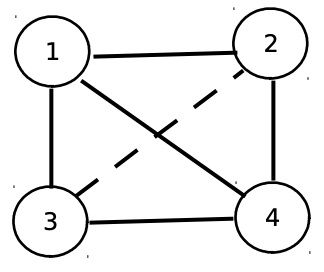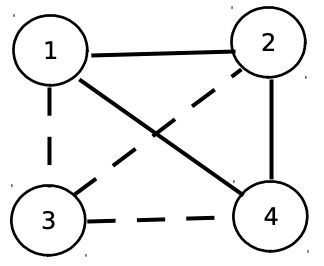시간 제한메모리 제한제출정답맞힌 사람정답 비율
2 초 512 MB62266.667%

## 문제

Lora is playing an online puzzle game. She receives an undirected graph with N vertices, numbered from 1 to N. The graph is such that between every two distinct vertices there is an edge, colored either in blue, or in red. We will call the graph redconnected, if from any vertex we can reach any other vertex, using only red edges. Similarly, a graph is blue-connected if from any vertex we can reach any other vertex, using only blue edges. We now define the state of the graph as a pair of numbers (A, B), such that:

• A=1 if the graph is red-connected, and A=0 if it is not
• B=1 if the graph is blue-connected, and B=0 if it is not

So for example, state (1, 0) describes a graph that is red-connected, but not blueconnected.

With a single click on a given edge, Lora can change its color (from blue to red or from red to blue). The goal of the game is, given an initial graph and a desired final state, to change the initial graph to one that is in the final state, using minimum amount of clicks (see the sample test cases for more information). Your task is to help Lora by writing a program colorgraph that finds the minimum amount of clicks needed to solve the problem.

## 입력

On the first line of the standard input is the positive integer N – the amount of vertices in the graph. After that, N lines follow, each with N space-separated numbers, describing the colors of the edges. Let us denote the j-th number on the i-th of those lines by Gij. If Gij=0, then the edge between i and j is red, and if Gij=1, then the edge between i and j is blue. It is guaranteed that Gij=Gji. For i=j, the value of Gij is irrelevant, since the graph contains no loops. On the last line are two space-separated numbers – A and B, describing the desired final state of the graph.

## 출력

If it is impossible to transform the graph into one with the desired state, you must print -1 on a single line of the standard output. In all other cases, on the first line of the standard output you must print a single integer K – the minimum amount of clicks Lora needs to make in order to transform the graph into the desired state. On each of the next K lines you must print a pair of numbers – the two endpoints of the edge that Lora should click on. If there is more than one solution, print any of them. The order, in which the edges are printed, as well as the order of the two endpoints, is irrelevant.

• 3 ≤ N ≤ 250

## 서브태스크

번호배점제한
115

N ≤ 7

235

The desired final state is (1, 1).

350

The desired final state is not (1, 1).

## 예제 입력 1

4
1 0 0 0
0 0 1 0
0 1 1 0
0 0 0 0
0 1


## 예제 출력 1

2
1 3
4 3


## 예제 입력 2

3
0 1 1
1 0 0
1 0 0
1 1


## 예제 출력 2

-1


## 예제 입력 3

3
0 1 1
1 0 0
1 0 0
0 1


## 예제 출력 3

0


## 힌트

The red edges are given with solid lines, while the blue ones are given with dotted lines.

In the first sample, we have the following initial graph in state (1, 0):After the change of edges 1-3 and 4-3, the graph is in the desired final state (0, 1) and looks as follows:In the second sample case, a graph with 3 vertices and state (1, 1) does not exist.

In the third sample case, the graph is already in the desired final state initially.

## 채점 및 기타 정보

• 예제는 채점하지 않는다.